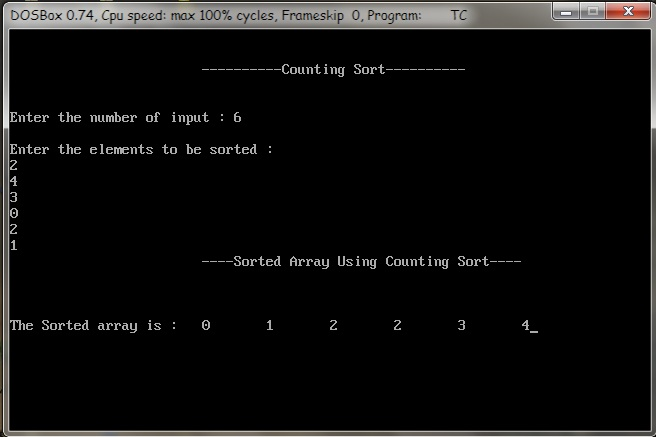Data Structure

# Counting sort

Counting sort is a linear time sorting algorithm used to sort items when they belong to a fixed and finite set. Integers which lie in a fixed interval, say k1 to k2, are examples of such items.

### Algorithm

```
COUNTING-SORT(A,B,k)

1. let C[0..k] be a new array

2. for i = 0 to k

3. 		C[i] = 0

4. for j = 1 to A.length

5. 		C[A[j]] = C[A[j]] + 1

6. // C[i] now contains the number of elements equal to i .

7. for i = 1 to k

8. 		C[i] = C[i] + C[i - 1]

9. // C[i] now contains the number of elements less than or equal to i .

10.for j = A.length downto 1

11.		B[C[A[j]]] = A[j]

12.		C[A[j]] = C[A[j]] - 1

```

### Counting sort Implementation

```
#include <stdio.h>

#include <conio.h>

void Counting_sort(int [], int, int);

void main()

{

int n,i,k=0,A;

clrscr();

printf("\n\n

\t\t\t----------Counting Sort----------\n\n\n");

printf("Enter the number of input : ");

scanf("%d",&n);

printf("\nEnter the elements to be sorted :\n");

for (i=1;i<=n;i++)

{

scanf("%d",&A[i]);

if(A[i] > k)

{

k = A[i];

}

}

Counting_sort(A, k, n);

getch();

}

void Counting_sort(int A[], int k, int n)

{

int i, j;

int B, C;

for(i = 0; i <= k; i++)

C[i] = 0;

for(j =1; j <= n; j++)

C[A[j]] = C[A[j]] + 1;

for(i = 1; i <= k; i++)

C[i] = C[i] + C[i-1];

for(j = n; j >= 1; j--)

{

B[C[A[j]]] = A[j];

C[A[j]] = C[A[j]] - 1;

}

printf("\t\t\t----Sorted Array Using Counting Sort----\n\n\n" );

printf("\nThe Sorted array is : ");

for(i=1;i<=n;i++)

{

printf("\t");

printf("%d",B[i]);

}

}```

### Output